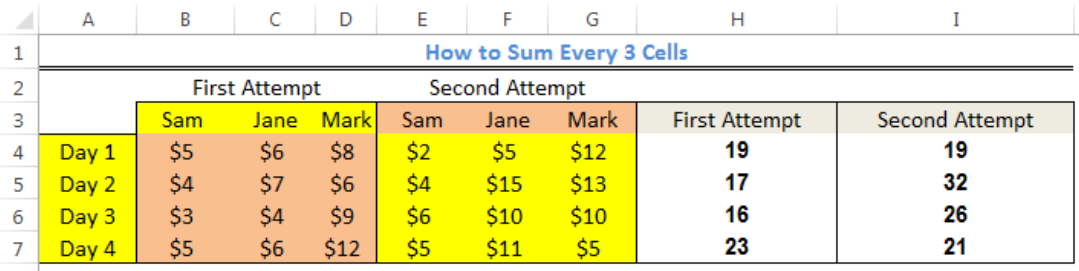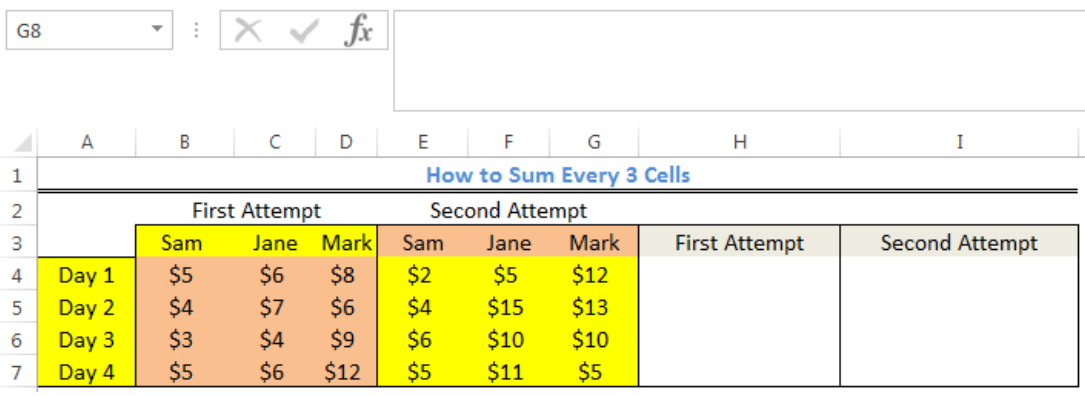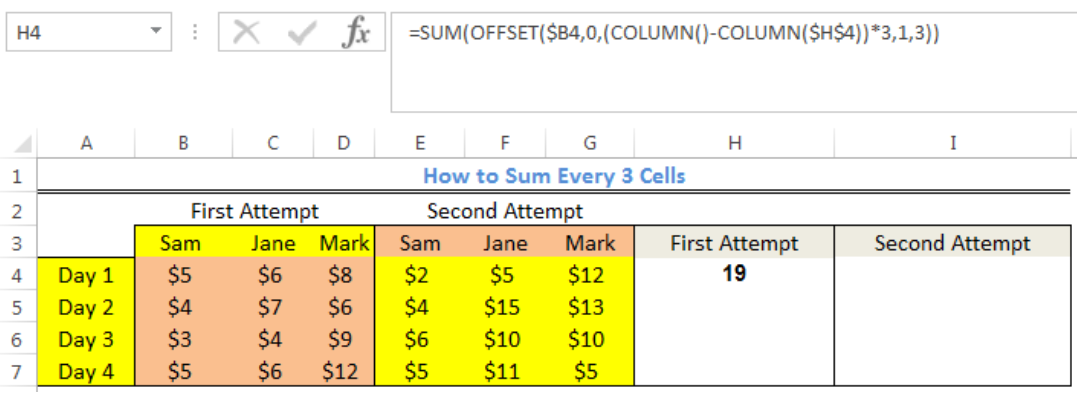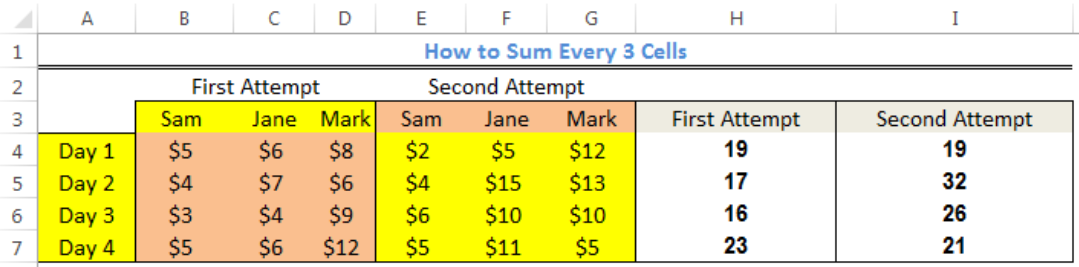Get instant live expert help with Excel or Google Sheets“My Excelchat expert helped me in less than 20 minutes, saving me what would have been 5 hours of work!”

#### Post your problem and you'll get expert help in seconds

Your message must be at least 40 characters
Our professional experts are available now. Your privacy is guaranteed.

# How to Sum Every 3 Cells

We can use the OFFSET function to sum a range of rows and columns from a reference cell. The OFFSET function eliminates the need for entering the desired range manually and the need for a complex formula. The steps below will walk through the process.Figure 1: How to Sum Every 3 Cells

## Syntax

`=SUM(OFFSET(REFERENCE, ROWS, COLUMN(S), HEIGHT, WIDTH))`

## Formula

`=SUM(OFFSET(\$B4,0,(COLUMN()-COLUMN(\$H\$4))*3,1,3))`

## Setting up the Data

We will set up the data as shown in figure 2. The data contains profit from sales trial of three salespersons for four days.Figure 2: Setting up the Data

## Sum Every 3 Cells

• Our goal is to sum the daily profit of the first and second attempt of the three salespersons.
• We will click on Cell H4
• We will insert the formula below and press enter
`=SUM(OFFSET(\$B4,0,(COLUMN()-COLUMN(\$H\$4))*3,1,3))`Figure 3: Sum of Cell B4, Cell C4, and Cell D4

• We will click on Cell H4
• We will use the fill handle (the small square box at the bottom right of the cell) to copy the formula to the other cells. First, drag the fill handle to Cell I4. Once the result displays, with Cell H4 still highlighted, drag down to the other cellsFigure 4: Sum of Every 3 Cells

## Explanation

`=SUM(OFFSET(\$B4,0,(COLUMN()-COLUMN(\$H\$4))*3,1,3))`

The OFFSET function delivers a range of 3 cells to the SUM function which returns the SUM of the cells.

For the OFFSET function, the first cell (Cell B4) serves as the reference. We enter this reference as a mixed reference with a locked column and relative row. 0 is used as the row number because we are not changing rows. The expression below is used for the COLUMN:

`(COLUMN()-COLUMN(\$H\$4))*3`

The formula detects the number of columns to OFFSET beginning from the reference cell. The offset for Cell H4 is 0 and Cell I4 is 3.

Because we want to SUM a 1 x 3 range of cells, we will put the height as 1 and the width as 3.

Finally, height is inputted as 1 and width is inputted as 3 since in this case we always want a 1 x 3 range of cells.

## Instant Connection to an Expert through our Excelchat Service

Most of the time, the problem you will need to solve will be more complex than a simple application of a formula or function. If you want to save hours of research and frustration, try our live Excelchat service! Our Excel Experts are available 24/7 to answer any Excel question you may have. We guarantee a connection within 30 seconds and a customized solution within 20 minutes.

### Did this post not answer your question? Get a solution from connecting with the expert.Another blog reader asked this question today on Excelchat:
Related blogs## Subscribe to Excelchat.coAnother blog reader asked this question today on Excelchat: